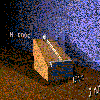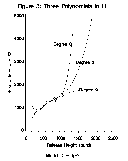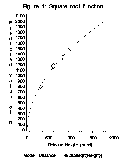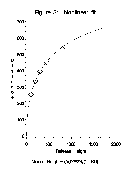# Teaching Statistics with Data of Historic Significance: Galileo's Gravity and Motion Experiments

David A. Dickey and J. Tim Arnold
North Carolina State University

Journal of Statistics Education v.3, n.1 (1995)

Copyright (c) 1995 by David A. Dickey and J. Tim Arnold, all rights reserved. This text may be freely shared among individuals, but it may not be republished in any medium without express written consent from the authors and advance notification of the editor.

Key Words: Galileo; Polynomial regression; Ockham's razor; Nonlinear regression.

## Abstract

This article demonstrates the use of two datasets as an aid in teaching polynomial and nonlinear regression. The data were gathered by Galileo during his studies of falling bodies and projectiles. In analyzing and discussing these data, students are challenged to give thought to parsimony, independent and dependent variables, and the importance of understanding the scientific nature of the experiment. The opportunities for class discussion are especially rich in this understandable and real experiment, particularly when coupled with graphical analysis.

# 1. Introduction

1 This article demonstrates the use of two very small datasets as an aid in learning polynomial and nonlinear regression. Students are mildly interested in this data at the outset because of its historical significance; their interest deepens as they follow, through discussion and analysis, Galileo's own thought pattern of 400 years ago. These data can lead students to an understanding not only of the statistician's technique, but also of some pitfalls that await the unwary. These lessons may be brought to light in directed discussions while teaching the two techniques of polynomial and nonlinear regression.

2 In the case of teaching polynomial regression, the first dataset provides an excellent example of the ease with which one's thinking can become confused during an analysis. That is, with this particular type of data it is quite important to think through the modelling procedure so the analysis is both statistically and scientifically correct. An example published in recent years (using these very data!) is referenced here, and helps to underscore the importance of such critical thinking while performing an analysis. The second dataset is used as an aid in learning nonlinear regression. The same lesson as before still applies--i.e., one must think carefully about the physical phenomenon that is being modelled.

# 2. The History of the Data

3 Galileo was 31 years old, lecturing at the University of Padua, when he began conducting a series of experiments and writing a treatise concerning the path of projectiles. While his writings and instruments came to be used primarily by gunners and cannoneers, the questions he posed were significant problems in physics. He was 42 years old when he completed these studies, at last giving an explicit formulation for the motion of falling bodies.

## 2.1. How the Experiment Was Performed

4 In studying the paths of projectiles, Galileo performed a series of experiments to provide different ways of looking at the question. We will look at two of these experiments. The primary experiment was performed with an inclined ramp elevated a certain height from the floor and a ball that served as the projectile. The ball was released at a set height on the ramp and allowed to roll down a groove set into the ramp. When the ball left the ramp's edge it began its descending flight at a speed depending on the release height. The measurement of release height (H) and distance traveled (D) before landing were recorded. The units of measurement are punti (points), and the data are shown in Table 1a.

5 In a related experiment, Galileo collected the data shown in Table 1b. The ball and the ramp were identical, but in this experiment a horizontal shelf was set into the end of the ramp. When the ball reached the edge of the inclined plane, it crossed the shelf before starting its fall (see Figure 1). Galileo realized that the ball in the first experiment was not merely falling to the ground when it left the ramp, but had an initial velocity in that direction simply due to the inclination of the ramp. His use of the shelf in this experiment allowed the ball to enter a state of freefall with zero vertical velocity because it cancelled out the downward velocity component of the ball during its roll down the ramp. Notes taken by Galileo for this experiment are shown in Figure 2. Because the data shown in Table 1a came from the experiment that used the ramp only, and the data in Table 1b came from the experiment using both ramp and shelf, we will refer to them as the ramp data (Table 1a) and the shelf data (Table 1b).Figure 1 (500K Mpeg Movie)

Figure 1. A Simulation of Galileo's Rolling Ball Experiment; Ramp with Horizontal Shelf.

```   Table 1a:  Galileo's Rolling Ball Data:  Ramp Only

Horizontal
Distance     Release Height
Traveled (D)   Above Table (H)
573           1000
534            800
495            600
451            450
395            300
337            200
253            100

Table 1b:  Galileo's Rolling Ball Data:  Ramp + Shelf

Horizontal
Distance     Release Height
Traveled (D)   Above Table (H)
1500           1000
1340            828
1328            800
1172            600
800            300

Source:  Drake, S. (1978), Galileo at Work, Chicago:
University of Chicago Press.
```Figure 2 (12K gif)

Figure 2. Galileo's Notes on the Rolling Ball Experiment.

## 2.2. Why Were the Experiments Done?

6 Galileo may have devised these experiments for two reasons. They were initiated during a time in Galileo's life when he was interested in the nature of falling bodies. Some students will recall the story of Galileo's claim that in a vacuum a cannonball and a feather, both dropped from the same height, would hit the floor at the same time. With the observational data available at that time, you can imagine the reception that idea received! In fact, Galileo was brought before the Spanish Inquisition and forced to say his ideas were wrong, then put under house arrest in his villa. In any case, one reason for these particular experiments may have been to provide evidence for Galileo's restricted inertial concept. While he had not formally declared that motion once imparted would remain uniform in speed, this experiment could provide such evidence. Another reason may have been to study the independent composition of two different motions (horizontal, imparted velocity and vertical velocity due to gravity).

# 3. Thinking Correctly about Nature

7 In 1980, the physicist/statistician team of Jeffreys and Berger (1992) resurrected Galileo's data (using the ramp data) and fit several models in order to make some points about Ockham's razor. Ockham's razor, sometimes known as the law of parsimony, is simply a maxim that states that simple explanations are usually better than complicated ones. In their article in the American Scientist, Jeffreys and Berger look at models for the horizontal distance of flight in the air (D) as polynomial functions of the release height (H). They point out that without the law of parsimony, Galileo might have chosen a more complex model than the quadratic model, if he only considered empirical measures of fit.

8 In one illustration, Jeffreys and Berger fit distance as a quadratic function in height and in another as a degree 6 polynomial in height to point out the usefulness of reducing model complexity. This type of modeling is logical if we look at how the experiment was run. The heights are given the role of the independent variable since they were chosen and fixed by Galileo and the distances were observed as a response to height.

9 We have followed the path taken by Jeffreys and Berger, but applying their technique to the shelf data from Table 1b. Graphs of the fitted functions (Figure 3) show some quite unbelievable results. The important point made by Jeffreys and Berger concerning parsimony and the fact that the degree 6 polynomial gives an exact fit can stimulate a rewarding discussion in the classroom. Both the cubic and degree 6 equations imply that as the release height increases, the distance traveled increases at a decreasing rate for a while, then beyond a certain height, distance increases at an accelerated rate--a phenomenon that is at best suspicious.Figure 3 (5.7K gif)

Figure 3. Three Polynomials in H; Model D = f(H). Three polynomial curves are fit to the shelf data to model distance (D) as a function of release height (H).

10 However, a closer look at this figure reveals that even when we simplify to the quadratic our model remains incorrect. Although the most parsimonious, the quadratic model predicts that there is a release height at which the distance traveled is maximized. For higher releases, the distance traveled begins to decrease until at some high release point, the ball comes speeding down the ramp only to drop immediately to the ground with no horizontal travel at all, not to mention what happens at even higher releases!

## 3.1. What Has Happened Here?

11 We have a situation that students can see at once is ridiculous. The problem, which discussion brings to light, is two-fold. For one thing, we are obviously extrapolating an empirically-fitted model beyond the range of observations on the independent variable, and are paying the price by obtaining predictions of behavior that contradict experience and common sense.

12 Secondly, and perhaps the more important lesson to be learned with this example, we are ignoring the science and just doing empirical approximation with polynomials. Galileo's laws of motion in a vacuum on a frictionless ramp indicate a quadratic relationship between distance and release height, but it is the height that should be a quadratic function of distance, not vice versa.

## 3.2. The Correct Approach (Finally!)

13 In the classroom, discussion at this point is most rewarding. The students are led to rethink the physical situation, and a few graphs are drawn on the board (or computer display). The resulting discussion will bring to light that if distance is on the vertical axis and height on the horizontal, the resulting quadratic equation that would make sense physically would give a parabola with a horizontal axis. If the parabola's axis were located at D = 0 (as seems logical, implying that releasing the ball at a height of zero would result in zero distance traveled) then distance would be a monotone increasing function of height but with the rate of increase falling off as height increases.

14 Now we regress distance on the square root (SH) of height (H). We first regress D on SH leaving in the intercept term as a check on our hypothesis; it should, of course, be zero. The analysis now makes sense--we fail to reject the hypothesis that the intercept is different from zero and go to the next stage of regressing D on SH, this time excluding the intercept term. This last analysis of the data now seems acceptable. It provides us with a good fit to the observed data points (Figure 4) and also an explanation of a natural phenomenon that agrees with common sense. Arriving at this final stage requires thinking about parsimony, assessing which variable is independent and which dependent, the direction in which the quadratic law applies for this physical situation, and the knowledge of how to perform polynomial regression.Figure 4 (6.3K gif)

Figure 4. Square Root Function. The curve shows the final correct fit for the shelf data: Distance = 47.086(sqrt (Height)).

# 4. Further Curiosities

15 Going on now to the situation for nonlinear regression, we turn to the data given in Table 1a (the ramp data). Here the ball is affected by the downward force of gravity and its own momentum down the inclined ramp. This dataset requires a quite different approach to modelling.

16 Attempting to analyze this dataset by using the polynomial model obtained from the shelf data (Table 1b) is an informative and rewarding exercise for the students that can also lead to a good classroom discussion. In fact, we note in passing that a figure accompanying the Jeffreys and Berger article would lead one to assume that the researchers were analyzing the data from the experiment using the shelf at the end of the ramp, i.e., the shelf data from Table 1b. We mention this as a warning signpost to the student: error surrounds us on all sides. Perhaps the point to this portion of the story is that we should be ever skeptical and alert with our data, no matter its source.

17 The dataset actually analyzed in that article was, however, the ramp dataset shown in Table 1a. With no horizontal shelf to neutralize the vector of initial velocity of the ball, we are left with a dataset too complicated for our original polynomial model. In fact, in an erratum to their _American Scientist_ article, Jeffreys and Berger (1992) state that the model should in fact be:

``` H = (A(D^2))/(1 + BD) (1) ```

where A and B are the parameters to be determined. Note that when D approaches -1/B, the denominator approaches zero, and hence the corresponding height approaches infinity. This case gives us the so-called `terminal distance' of D = -1/B. That there can be such a thing as a terminal or limiting distance that the ball may travel should be evident from the physical experiment itself: the ball cannot possibly travel further than that distance allowed by the ramp's inclination, i.e., the point at which an imaginary extension of the ramp would touch the floor. In the earlier experiment, there was no terminal distance because the horizontal shelf did away with this limitation.

18 So the problem now is to estimate these two parameters A and B. Using nonlinear regression to fit the model (1) above, we see that the fit is very sensitive to the initial values for A and B. Several of our attempts to take initial values from the model already fitted resulted in failure to converge. To get good initial values, take the values for H and D from Galileo's highest release point and substitute them into the equation above to get A as a function of B, thereby reducing the function to one parameter (B) and forcing the fitted function through the rightmost datapoint. Searching over B gives a good fit, and this B with its associated A = f(B) were used as initial values in a two parameter nonlinear regression, resulting in a very good fit.

19 Note, however, that in modelling the equation provided in (1) we have misassigned the roles of dependent and independent variables. A final model was fit by solving (1) for D as a function of H. In other words, we algebraically manipulated (1) to produce a quadratic function in D, set this equal to zero and applied the formula for roots of a quadratic, being sure to take the positive root. The nonlinear regression analysis returns a very small error mean square 6.65. The model implies a terminal distance 1/.0011264789 = 888 punti. Figure 5 shows a graph of the nonlinear fitted function. We note in the conclusion of this analysis that physics theory implies that the theoretical values for A and B are particular functions of the ramp inclination angle and table height, so that both of these could be estimated from the data.Figure 5 (4.9K gif)

Figure 5. Nonlinear Fit. The curve shows the correct fit for the ramp data: Height = (A(D^2))/(1 = BD).

# 5. Conclusion

20 The opportunities for class discussion are especially rich in this understandable and real experiment, particularly when analyzing Figure 3. Ultimately we find that the combination of appropriate statistical and subject matter knowledge, graphing of the results, and a degree of skepticism have brought us to a model that fits the data quite well. Galileo has provided us with two datasets from which we can learn both the technique of modelling and the dangers involved in analyzing data without considering the underlying nature of the world.

# 6. Getting the Data

21 The file galileo.dat.txt contains the raw data. The file galileo.txt is a documentation file containing a brief description of the dataset.

# Appendix - Key to Variables in galileo.dat.txt

Columns
1 - 4 Horizontal distance traveled
6 - 9 Release height above table

Values are aligned and delimited by blanks. The two datasets are separated in the file by a row of zeros.

# References

Drake, S. (1978), Galileo at Work, Chicago: University of Chicago Press.

Jeffreys, W. H., and Berger, J. O. (1992), "Ockham's Razor and Bayesian Analysis," American Scientist, 80, 64-72 (Erratum, p. 116).

### Erratum

Figure 4 shows the correct model. The original figure and caption reported an incorrect model. The error was reported by Dominick Latour of SAS Institute. (Corrected April 18, 1995)

David A. Dickey

dickey@stat.ncsu.edu
J. Tim Arnold
arnold@stat.ncsu.edu
Department of Statistics, Box 8203
North Carolina State University
Raleigh, NC 27695-8203